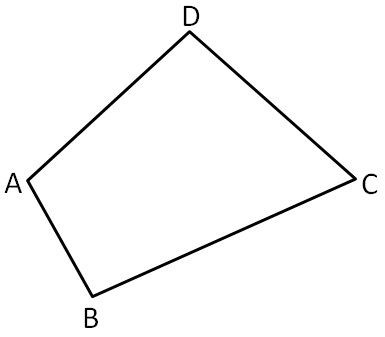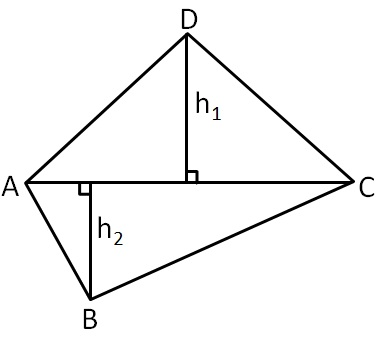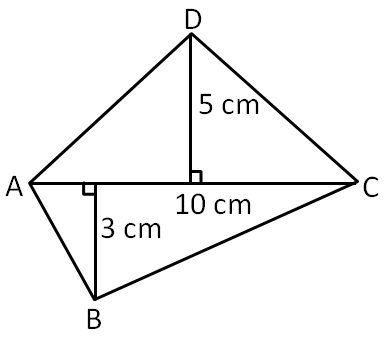Chapter 9 Class 8 Mensuration
Concept wise

To find area of any general quadrilateral,

We split it into triangles and add their area parallelogram has opposites sides equal & parallelWe join ACSo, we have two triangles - Δ ADC and Δ ABC

Let h 1 be height from point D to AC

& h 2 be height from point B to AC

So,

= Area of Δ ADC + Area of Δ ABC

= 1/2 × AC × h 1 + 1/2 × AC × h 2

= 1/2 × AC × (h 1 + h 2 )

= 1/2 × (h 1 + h 2 )

where d = Length of DiagonalGiven

Length of diagonal = d = 10 cm

Length of Perpendicular dropped on AC

h 1 = 5 cm

& h 2 = 3 cm

Now,

Area of ABCD = d/2 (h 1 + h 2 )

= 10/2 (5 + 3)

= 10/2 × 8

= 5 × 8

= 40 cm 2

Area of quadrilateral = 40 cm 2

Learn in your speed, with individual attention - Teachoo Maths 1-on-1 Class